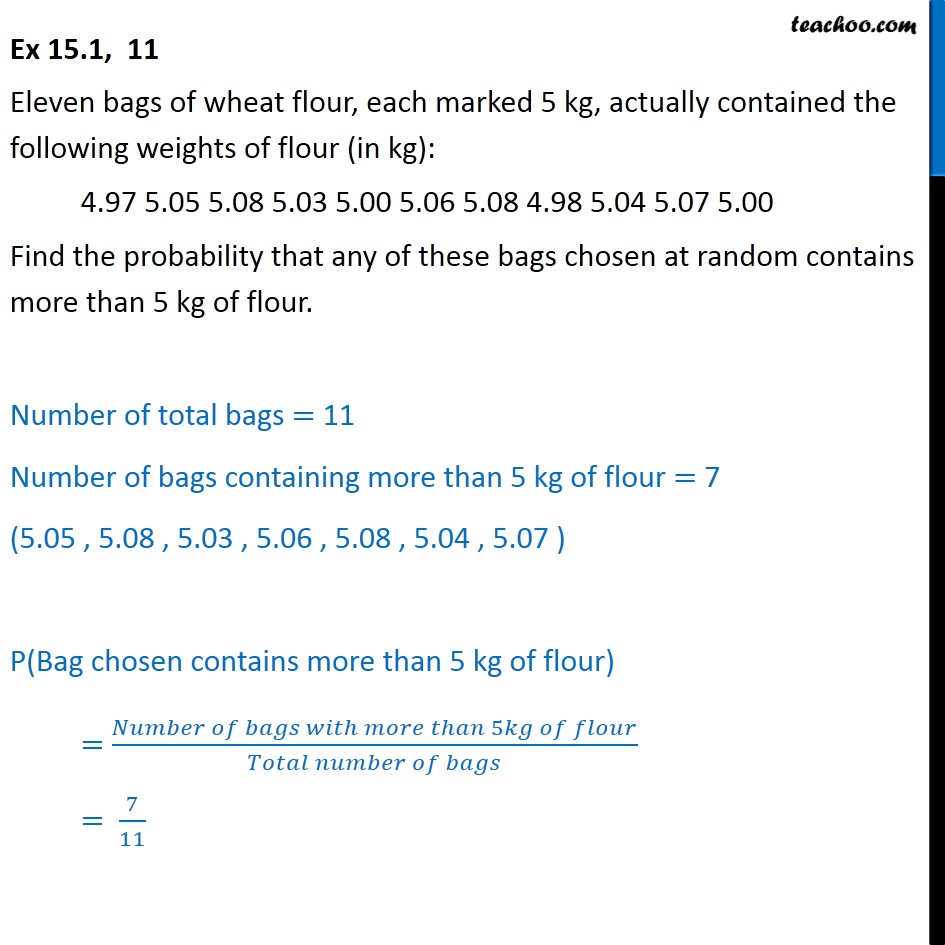1. Chapter 15 Class 9 Probability
2. Serial order wise
3. Ex 15.1

Transcript

Ex 15.1, 11 Eleven bags of wheat flour, each marked 5 kg, actually contained the following weights of flour (in kg): 4.97 5.05 5.08 5.03 5.00 5.06 5.08 4.98 5.04 5.07 5.00 Find the probability that any of these bags chosen at random contains more than 5 kg of flour. Number of total bags = 11 Number of bags containing more than 5 kg of flour = 7 (5.05 , 5.08 , 5.03 , 5.06 , 5.08 , 5.04 , 5.07 ) P(Bag chosen contains more than 5 kg of flour) = (𝑁𝑢𝑚𝑏𝑒𝑟 𝑜𝑓 𝑏𝑎𝑔𝑠 𝑤𝑖𝑡ℎ 𝑚𝑜𝑟𝑒 𝑡ℎ𝑎𝑛 5𝑘𝑔 𝑜𝑓 𝑓𝑙𝑜𝑢𝑟)/(𝑇𝑜𝑡𝑎𝑙 𝑛𝑢𝑚𝑏𝑒𝑟 𝑜𝑓 𝑏𝑎𝑔𝑠) = 7/11

Ex 15.1

Chapter 15 Class 9 Probability
Serial order wise